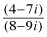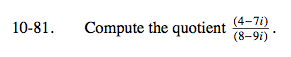### Home > A2C > Chapter 10 > Lesson 10.2.2 > Problem10-81

10-81.

Compute the quotient. Homework Help ✎$\frac{(4-7i)}{(8-9i)}\cdot \frac{(8+9i)}{(8+9i)}$

$\frac{19-4i}{29}=\frac{19}{29}-\frac{4}{29}i$